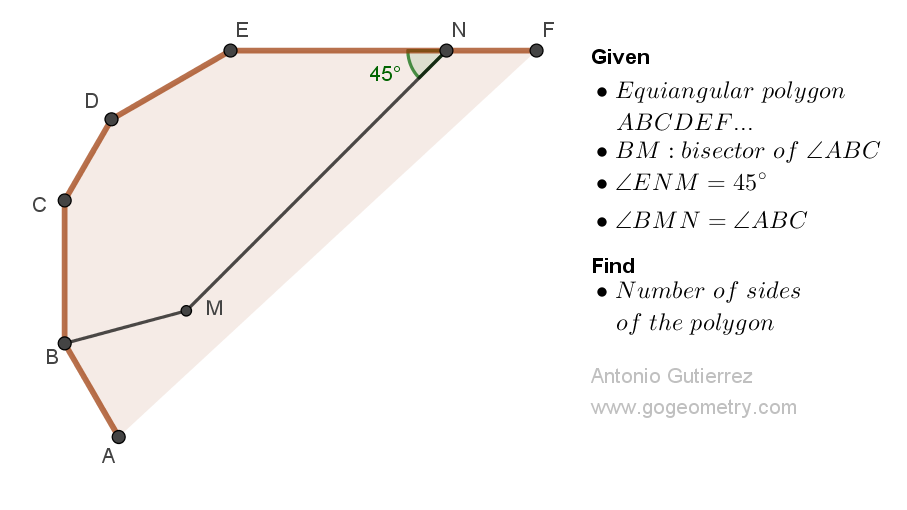## Friday, March 3, 2023

### Geometry Problem 1518: Boost Your Geometry Skills: Solve for the Number of Sides in an Equiangular Polygon with an Interior Point and Bisected Angle

Geometry Problem 1518. Post your solution in the comment box below.
Level: Mathematics Education, K-12 School, Honors Geometry, College.

Details: Click on the figure below.More Details

To post a solution to this problem click Comment underneath the post, or click into the line that says, “Enter Comment.” Type what you want to say and press Publish to post your solution.

1.2.Let <ABM = <CBM =p then in hexagon BCDENM
p + 2p + 2p + 2p + 45 + 2p = 720
So p = 75, each internal angle of the polygon = 150 and thus each exterior angle = 30

Hence the number of sides of the polygon = 360/30 = 12
So the polygon is 12-sided

Sumith Peiris
Moratuwa
Sri Lanka